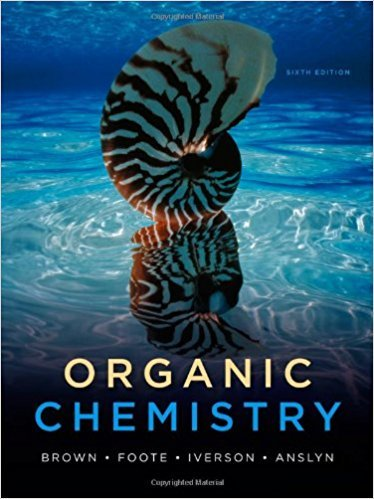×

> > Organic Chemistry 6

# Organic Chemistry 6th Edition - Solutions by Chapter## Full solutions for Organic Chemistry | 6th Edition

ISBN: 9780840054982Organic Chemistry | 6th Edition - Solutions by Chapter

Solutions by Chapter
4 5 0 337 Reviews
##### ISBN: 9780840054982

This expansive textbook survival guide covers the following chapters: 29. The full step-by-step solution to problem in Organic Chemistry were answered by , our top Chemistry solution expert on 09/09/17, 04:06AM. Organic Chemistry was written by and is associated to the ISBN: 9780840054982. Since problems from 29 chapters in Organic Chemistry have been answered, more than 23648 students have viewed full step-by-step answer. This textbook survival guide was created for the textbook: Organic Chemistry, edition: 6.

Key Chemistry Terms and definitions covered in this textbook
• antioxidants

Radical scavengers that prevent autooxidation by preventing radical chain reactions from beginning.

• aqueous solution

A solution in which water is the solvent. (Chapter 4: Introduction)

• bidentate ligand

A ligand in which two linked coordinating atoms are bound to a metal. (Section 23.3)

• bond order

The number of bonding electron pairs shared between two atoms, minus the number of antibonding electron pairs: bond order = (number of bonding electrons - number of antibonding electrons)/2. (Section 9.7)

• Conformation

Any three-dimensional arrangement of atoms in a molecule that results from rotation about a single bond.

• double bond

A covalent bond involving two electron pairs. (Section 8.3)

• elementary reaction

A process in a chemical reaction that occurs in a single step. An overall chemical reaction consists of one or more elementary reactions or steps. (Section 14.6)

• equilibrium-constant expression

The expression that describes the relationship among the concentrations (or partial pressures) of the substances present in a system at equilibrium. The numerator is obtained by multiplying the concentrations of the substances on the product side of the equation, each raised to a power equal to its coefficient in the chemical equation. The denominator similarly contains the concentrations of the substances on the reactant side of the equation. (Section 15.2)

• geminal

Two groups connected to the same carbon atom. For example, a geminal dihalide is a compound with two halogens connected to the same carbon atom.

• haloalkane

An organic compound containing at least one halogen.

• Heat of reaction (DH0 )

The difference in enthalpy between reactants and products. If the enthalpy of products is lower than that of the reactants, heat is released and the reaction is exothermic. If the enthalpy of the products is higher than that of the reactants, energy is absorbed, and the reaction is endothermic

• Heterocyclic aromatic amine

An amine in which nitrogen is one of the atoms of an aromatic ring.

• Hydration

• internal energy

The total energy possessed by a system. When a system undergoes a change, the change in internal energy, ?E, is defined as the heat, q, added to the system, plus the work, w, done on the system by its surroundings: ?E = q + w. (Section 5.2)

• lecithins

Phosphoglycerides thatcontain choline.

• melt transition temperature (Tm)

The temperature at which the crystallineregions of a polymer become amorphous.

• molecular equation

A chemical equation in which the formula for each substance is written without regard for whether it is an electrolyte or a nonelectrolyte. (Section 4.2)

• partial pressure

The pressure exerted by a particular gas in a mixture. (Section 10.6)

• photoelectric effect

The emission of electrons from a metal surface induced by light. (Section 6.2)

• retention of configuration

During a reaction, when the configuration of a chirality center remains unchanged.

×